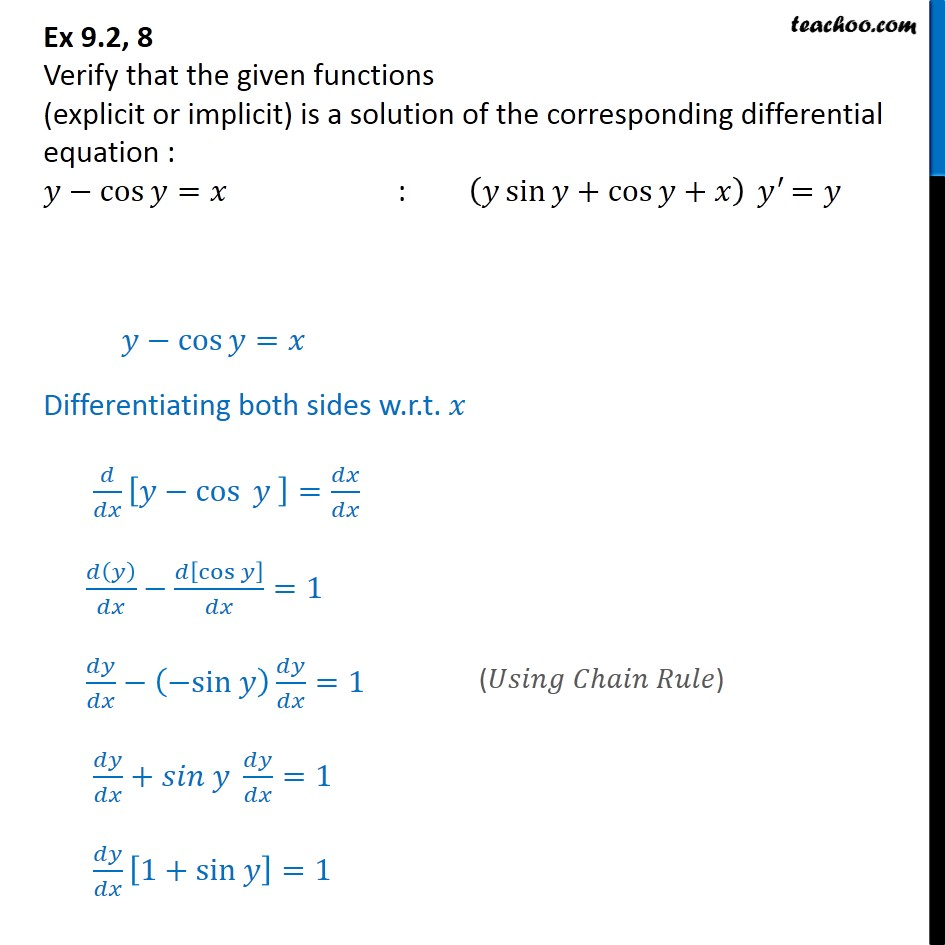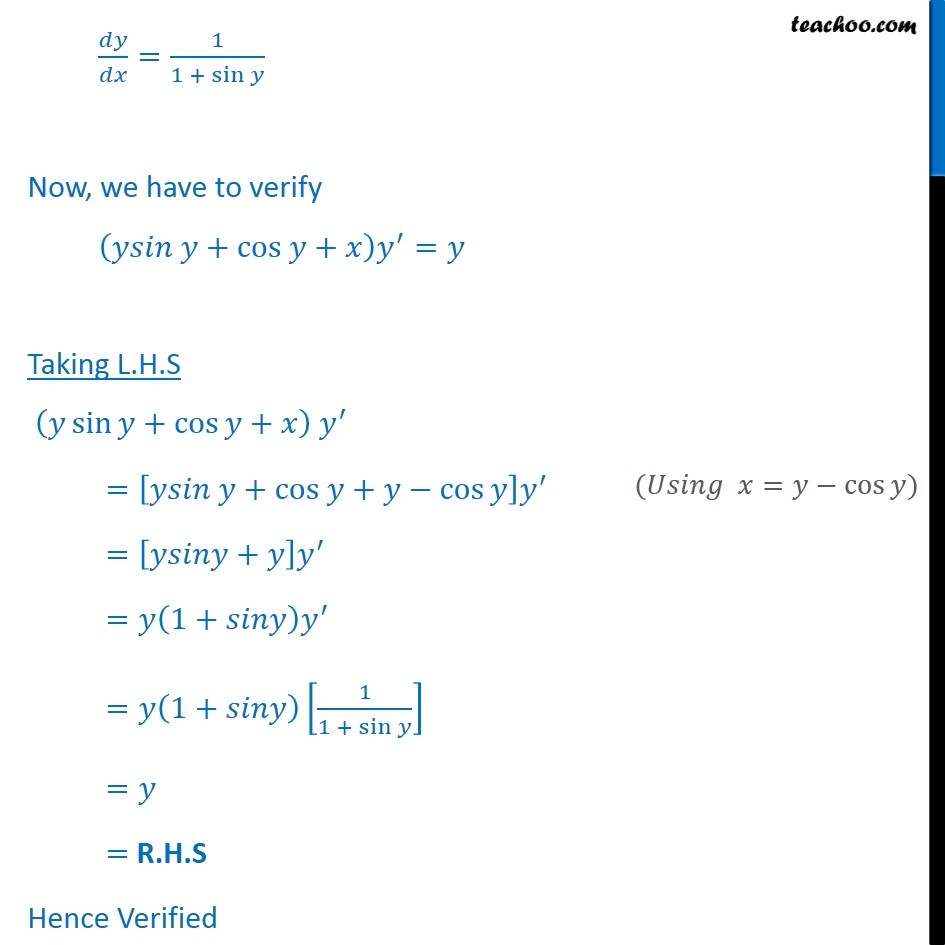Ex 9.2

Chapter 9 Class 12 Differential Equations
Serial order wiseLearn in your speed, with individual attention - Teachoo Maths 1-on-1 Class

### Transcript

Ex 9.2, 8 Verify that the given functions (explicit or implicit) is a solution of the corresponding differential equation : 𝑦−cos⁡〖𝑦=𝑥〗 : (𝑦 sin⁡〖𝑦+cos⁡〖𝑦+𝑥〗 〗 ) 〖 𝑦〗^′=𝑦 𝑦−cos⁡〖𝑦=𝑥〗 Differentiating both sides w.r.t. 𝑥 𝑑/𝑑𝑥 [𝑦−〖cos 〗⁡𝑦 ]=𝑑𝑥/𝑑𝑥 𝑑(𝑦)/𝑑𝑥−𝑑[cos 𝑦]/𝑑𝑥=1 𝑑𝑦/𝑑𝑥−(−sin 𝑦) 𝑑𝑦/𝑑𝑥=1 𝑑𝑦/𝑑𝑥+𝑠𝑖𝑛 𝑦 𝑑𝑦/𝑑𝑥=1" " 𝑑𝑦/𝑑𝑥 [1+sin 𝑦]=1 𝑑𝑦/𝑑𝑥=1/(1 + sin 𝑦) Now, we have to verify (𝑦𝑠𝑖𝑛 𝑦+cos 𝑦+𝑥) 𝑦^′=𝑦 Taking L.H.S (𝑦 sin⁡〖𝑦+cos⁡〖𝑦+𝑥〗 〗 ) 〖 𝑦〗^′ =[𝑦𝑠𝑖𝑛 𝑦+cos 𝑦+𝑦−cos⁡𝑦 ] 𝑦^′ =[𝑦𝑠𝑖𝑛𝑦+𝑦] 𝑦^′ =𝑦(1+𝑠𝑖𝑛𝑦) 𝑦^′ =𝑦(1+𝑠𝑖𝑛𝑦)[1/(1 + sin⁡𝑦 )] =𝑦 = R.H.S Hence Verified